Answer all questions and submit them either as an IPython notebook, LaTeX document, or Markdown document. Each question is worth 25 points.

This homework is due Tuesday, October 25, 2016.

In :
%matplotlib inline
import numpy as np
import pymc3 as pm
import pylab as plt
import pandas as pd

# Set seed
np.random.seed(10011)


# Question 1¶

Epidemiologists are interested in studying the sexual behavior of individuals at risk for HIV infection. Suppose 1500 gay men were surveyed and each was asked how many risky sexual encounters he had in the previous 30 days. Let $n_i$ denote the number of respondents reporting $i$ encounters, for $i = 1, \ldots , 16$. The DataFrame below contains these reponses:

In :
encounters = pd.DataFrame({'ecount': np.arange(17),
'freq': [379, 299, 222, 145, 109, 95, 73, 59,
45, 30, 24, 12, 4, 2, 0, 1, 1]})

encounters.freq.plot.bar()

Out:
<matplotlib.axes._subplots.AxesSubplot at 0x111cb60f0>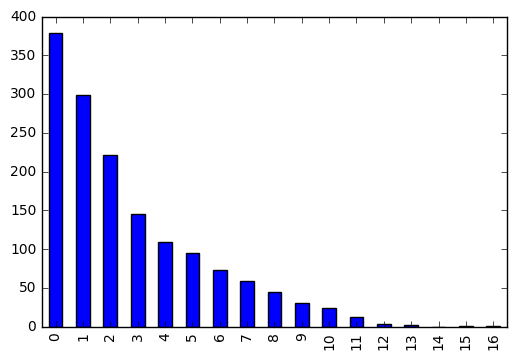These data are poorly fitted by a Poisson model. It is more realistic to assume that the respondents comprise three groups. First, there is a group of people who, for whatever reason, report zero risky encounters even if this is not true. Suppose a respondent has probability $\alpha$ of belonging to this group.

With probability $\beta$, a respondent belongs to a second group representing typical behavior. Such people respond truthfully, and their numbers of risky encounters are assumed to follow a $\text{Poisson}(\mu)$ distribution.

Finally, with probability $1 − \alpha − \beta$, a respondent belongs to a high-risk group. Such people respond truthfully, and their numbers of risky encounters are assumed to follow a $\text{Poisson}(\lambda)$ distribution.

The parameters in the model are $\alpha, \beta, \mu$ and $\lambda$. At the tth iteration of EM, we use $\theta^{(t)} = (\alpha^{(t)}, \beta^{(t)}, \mu^{(t)}, \lambda^{(t)})$ to denote the current parameter values. The likelihood of the observed data is given by:

$$L(\theta | n_0, \ldots, n_16) \propto \prod_{i=0}^{16} \left[ \frac{\pi_i(\theta)}{i!} \right]^{n_i}$$

where $\pi_i(\theta) = \alpha 1_{(i=0)} + \beta \mu^i \exp(-\mu) + (1-\alpha - \beta) \lambda^i \exp(-\lambda)$.

The observed data are in the encounters table above; the complete data may be construed to be $(n_{z,0}, n_{t,0}, n_{p,0}), \ldots, (n_{z,16}, n_{t,16}, n_{p,16})$, where $k = z, t, p$ correspond to zero, typical and promiscuous groups, respectively. That is, $n_0 = n_{z,0} + n_{t,0} + n_{p,0}$, and so on. Let $N = \sum_{i=0}^{16} n_i = 1500$.

Also define:

\begin{align} z_0(\theta) &=& \frac{\alpha}{\pi_0(\theta)} \\ t_i(\theta) &=& \frac{\beta \mu^i \exp(-\mu)}{\pi_i(\theta)} \\ p_i(\theta) &=& \frac{(1-\alpha-\beta)\lambda^i \exp(-\lambda)}{\pi_i(\theta)} \end{align}

which correspond to probabilities that respondents with $i$ risky encounters belong to various groups.

a. Show that the EM algorithm provides the following updates:

\begin{align} \alpha^{(t+1)} &=& \frac{n_0 z_0(\theta^{(t)})}{N} \\ \beta^{(t+1)} &=& \sum_i \frac{n_i t_i(\theta^{(t)})}{N} \\ \mu^{(t+1)} &=& \frac{\sum_i i n_i t_i(\theta^{(t)})}{\sum_i n_i t_i(\theta^{(t)})} \\ \lambda^{(t+1)} &=& \frac{\sum_i i n_i p_i(\theta^{(t)})}{\sum_i n_i p_i(\theta^{(t)})} \end{align}

b. Extimate the parameters of the model using the observed data.

### Part a¶

Recall that the E step involves obtaining the expected value of the latent values. In our case, what we need are the number of each behavior type for each encounter count. For example, the number of "typical" behaviour individuals for the 4-encounter data:

$$n_{t4} = n_4 t_4(\theta)$$

we are given the probabilites $z_i(\theta), t_i(\theta), p_i(\theta)$ above, so this is easy:

\begin{align} n_{z0} &=& n_0 z_0(\theta) \\ n_{ti} &=& \sum_i n_i t_i(\theta) \\ n_{pi} &=& \sum_i n_i p_i(\theta) \end{align}

And so, $n_z = n_{z0}, n_t = \sum_i n_{ti}$, and $n_p = \sum_i n_{pi}$.

Since the mixture proportions $\alpha$ and $\beta$ are multinomial, we there maximum likelihood values are known ($\hat{p} = x/N$), so:

$$\alpha^{(t+1)} = \frac{n_{z0}}{N} = \frac{n_0 z_0(\theta^{(t)})}{N}$$

as shown. Similarly, for $\beta$:

$$\beta^{(t+1)} = \frac{n_t}{N} = \frac{\sum_i n_{ti}}{N} = \frac{\sum_i n_i t_i(\theta^{(t)})}{N}$$

For the last two, these are Poisson parameters. We can solve for this:

\begin{align} L(\lambda) &=& \log \Pi_{i=1}^{n} f(x_i | \lambda) \\ &=& \sum_i \log \left[ \frac{e^{-\lambda} \lambda^{x_i}}{x_i!} \right] \\ &=& -n\lambda + \log(\lambda)\sum_i x_i - \sum_i \log(x!) \end{align}

$$\frac{dL(\lambda)}{d\lambda} = -n \frac{\sum_i x_i}{\lambda} = 0$$

which is just the mean, $\bar{x}$. So, for the mean of the data for the two Poisson populations:

$$\bar{x}_j = \frac{\sum_i y_{ij} x_i}{\sum_i y_{ij}}$$

where for $\mu$, $y_{ij} = n_i t_i(\theta)$ and for $\lambda$, $y_{ij} = n_i p_i(\theta)$. Plug these in, and you have the result.

$$\pi_i(\theta) = \alpha 1_{(i=0)} + \beta \mu^i \exp(-\mu) + (1-\alpha - \beta) \lambda^i \exp(-\lambda)$$

### Part b¶

In :
def calc_pi(θ, i):
α, β, μ, λ = θ
return α*(i==0) + β*(μ**i)*np.exp(-μ) + (1-α-β)*(λ**i)*np.exp(-λ)

In :
def e_step(x, θ):

α, β, μ, λ = θ

z = α/calc_pi(θ, 0)

t = [β*(μ**i)*np.exp(-μ)/calc_pi(θ,i) for i,x in encounters.iterrows()]

p = [(1-α-β)*(λ**i)*np.exp(-λ)/calc_pi(θ,i) for i,x in encounters.iterrows()]

return z, t, p

In :
def m_step(x, z, t, p):

i = x.ecount.values
n = x.freq.values
N = n.sum()

α = n*z/N

β = (n*t/N).sum()

μ = (i*n*t).sum() / (n*t).sum()

λ = (i*n*p).sum() / (n*p).sum()

return α, β, μ, λ

In :
def run_em(theta0, x=encounters):

# Initialize values
θ = theta0

# Stopping criterion
crit = 1e-5

# Convergence flag
converged = False

# Loop until converged
while not converged:

# E-step
z, t, p = e_step(x, θ)
# M-step
θ_new = m_step(x, z, t, p)

# Check convergence
converged = np.all(np.abs((np.array(θ_new) - np.array(θ)) < crit))
θ = θ_new

return θ

In :
run_em(theta0=(0.1, 0.1, 2, 3))

Out:
(0.1221533024160731,
0.56254176336233563,
1.4673942597455256,
5.9387889066747022)

# Question 2¶

Suppose $y$ has a binomial distribution with parameters $n$ and $p$, and we are interested in the log-odds value $\theta = \log(p/(1 − p))$. Our prior for $\theta$ is that $\theta \sim N(\mu, \sigma^2)$. It follows that the posterior density of $\theta$ is given, up to a proportionality constant, by:

$$\pi(\theta | y) \propto \frac{\exp(y\theta)}{(1 + exp(\theta))^n} \exp\left[\frac{-(\theta − \mu)^2}{2\sigma^2}\right]$$

For example, suppose we are interested in learning about the probability that a possibly-biased coin lands heads when tossed. A priori we believe that the coin is fair, so we assign $\theta$ a $N(0,.25)$ prior. We toss the coin $n = 5$ times and obtain $y = 5$ heads.

1. Using a normal approximation to the posterior density, compute the probability that the coin is biased toward heads (i.e., that θ is positive).
2. Using the prior density as a proposal density, design a rejection algorithm for sampling from the posterior distribution. Using simulated draws from your algorithm, approximate the probability that the coin is biased toward heads.
3. Using the prior density as a proposal density, simulate values from the posterior distribution using the SIR algorithm. Approximate the probability that the coin is biased toward heads.
In :
n = 5
y = 5

In :
def calc_posterior(θ, y=y, n=n, μ=0, σ=0.25):
return  y*θ - n*np.log(1 + np.exp(θ)) - ((θ - μ)**2/(2*σ**2))

invlogit = lambda x: 1/(1+np.exp(-x))


### Part 1¶

In :
from scipy.optimize import minimize

calc_posterior_min = lambda *args: -calc_posterior(*args)

init_value = -1

opt = minimize(calc_posterior_min, init_value, method='L-BFGS-B')
mode = opt.x
var = opt.hess_inv.todense()
mode, var

Out:
(array([ 0.14494609]), array([[ 0.05797844]]))
In :
norm.cdf(0, mode, np.sqrt(var))

Out:
0.2735977908690973

### Part 2¶

In :
from scipy.stats import norm

prior = norm(0, 0.25)

In :
def calc_diff(theta, mu=0, sigma=0.25):

return calc_posterior(theta) - prior.logpdf(theta)

calc_diff_min = lambda *args: -calc_diff(*args)

In :
opt = minimize(calc_diff_min,
-1,
method='bfgs')

In :
c = opt.fun

In :
draws = 10000

In :
# Draw samples from g(theta)
theta = prior.rvs(size=draws)

# Calculate probability under g(theta)
gvals = prior.logpdf(theta)

# Calculate probability under f(theta)
fvals = calc_posterior(theta)

# Calculate acceptance probability
p = np.exp(fvals - gvals + c)

sample = theta[np.random.random(draws) < p]

In :
(invlogit(sample)<0.5).mean()

Out:
0.27493261455525608

### Part 3¶

Calculate importance weights

In :
w = np.exp(fvals - gvals - max(fvals - gvals))

Out:
array([ 0.10769292,  0.29650221,  0.08566229, ...,  0.10966467,
0.09353153,  0.24888339])
In :
p_sir = w/w.sum()

In :
theta_sir = theta[np.random.choice(range(len(theta)), size=10000, p=p_sir)]

In :
plt.hist(theta_sir);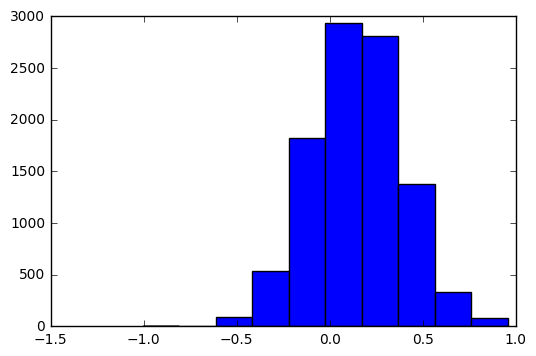In :
(theta_sir<0).mean()

Out:
0.2762

# Question 3¶

The goal of this problem is to investigate the role of the proposal distribution in a Metropolis-Hastings algorithm designed to simulate from the posterior distribution of the mixture parameter $\delta$.

1. Simulate 200 realizations from the mixture distribution: $$y_i \sim \delta N(7, 0.5^2) + (1-\delta) N(10, 0.5^2)$$ with $\delta = 0.7$. Plot a histogram of these data.
2. Implement an ABC procedure to simulate from the posterior distribution of $\delta$, using your data from part (1).
3. Implement a random walk M-H algorithm with proposal $\delta^{\prime} = \delta^{(i)} + \epsilon$ with $\epsilon \sim Unif(−1,1)$.
4. Reparameterize the problem letting $U = \log\left[\frac{\delta}{1 - \delta}\right]$ and $u^{\prime} = u^{(i)} + \epsilon$. Implement a random walk chain in U-space.
5. Compare the estimates and convergence behavior of the three algorithms.

In part (1), you are asked to simulate data from a distribution with $\delta$ known. For parts (2)–(4), assume $\delta$ is unknown with prior $\delta \sim Unif( 0,1)$. For parts (2)–(4), provide an appropriate plot and a table summarizing the output of the algorithm.

To facilitate comparisons, use the same number of iterations, random seed, starting values, and burn-in period for all implementations of the algorithm.

### Part 1¶

In :
# Sample data
n = 200
delta = 0.7

y = np.where(np.random.random(n) < delta, np.random.normal(7, 0.5, n), np.random.normal(10, 0.5, n))

In :
plt.hist(y)

Out:
(array([ 14.,  59.,  46.,  17.,   0.,   5.,  12.,  31.,  13.,   3.]),
array([  5.82439521,   6.38620264,   6.94801007,   7.5098175 ,
8.07162492,   8.63343235,   9.19523978,   9.75704721,
10.31885463,  10.88066206,  11.44246949]),
<a list of 10 Patch objects>)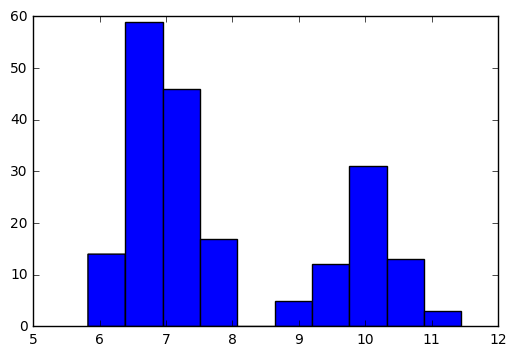### Part 2¶

In :
np.mean(y), np.std(y)

Out:
(7.9231652131648911, 1.5150267222378637)
In :
n_samples = 100
epsilons = [0.05, 0.01]

# List for holding accepted samples
samples = []

while len(samples) < n_samples:

# Simulate from prior
d = np.random.random()

# Simulate data
y_sim = np.where(np.random.random(n) < d, np.random.normal(7, 0.5, n), np.random.normal(10, 0.5, n))

if (np.abs(np.mean(y_sim) - np.mean(y)) < epsilons) & (np.abs(np.std(y_sim) - np.std(y)) < epsilons):

samples.append(d)

In :
plt.hist(samples)

Out:
(array([  1.,   4.,   8.,  13.,  23.,  16.,  19.,  10.,   4.,   2.]),
array([ 0.57736545,  0.59805126,  0.61873708,  0.63942289,  0.66010871,
0.68079452,  0.70148034,  0.72216616,  0.74285197,  0.76353779,
0.7842236 ]),
<a list of 10 Patch objects>)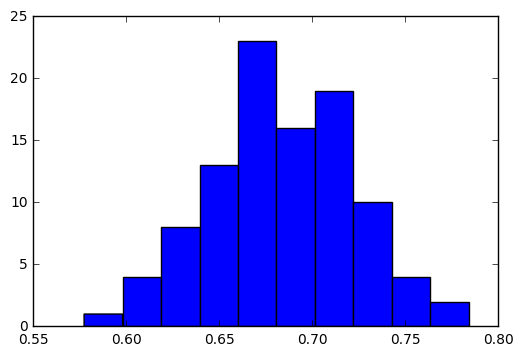### Part 3¶

In :
plt.hist(y)

Out:
(array([ 14.,  59.,  46.,  17.,   0.,   5.,  12.,  31.,  13.,   3.]),
array([  5.82439521,   6.38620264,   6.94801007,   7.5098175 ,
8.07162492,   8.63343235,   9.19523978,   9.75704721,
10.31885463,  10.88066206,  11.44246949]),
<a list of 10 Patch objects>)There are several ways of solving this; here is the easiest (though not the most general)

In :
from scipy.stats import norm
dnorm = norm.pdf

def calc_posterior(d):
return np.log(d*dnorm(y, 7, 0.5) + (1-d)*dnorm(y, 10, 0.5)).sum()

In :
n_iterations = 10000

# Initialize trace for parameters
trace = np.empty(n_iterations+1)

# Set initial values
trace = 0.5
# Calculate joint posterior for initial values
current_log_prob = calc_posterior(trace)

# Initialize acceptance counts
accepted = 0

for i in range(n_iterations):

if not i%1000: print('Iteration %i' % i)

# Grab current parameter values
d_current = trace[i]

# Propose new value for d
d_prop = d_current + np.random.uniform(-1, 1)
while (d_prop<0) or (d_prop>1):
d_prop = d_current + np.random.uniform(-1, 1)

# Calculate log posterior with proposed value
proposed_log_prob = calc_posterior(d_prop)

# Log-acceptance rate
alpha = proposed_log_prob - current_log_prob

# Test proposed value
if np.log(np.random.random()) < alpha:
# Accept
trace[i+1] = d_prop
current_log_prob = proposed_log_prob
accepted += 1
else:
# Reject
trace[i+1] = trace[i]

Iteration 0
Iteration 1000
Iteration 2000
Iteration 3000
Iteration 4000
Iteration 5000
Iteration 6000
Iteration 7000
Iteration 8000
Iteration 9000

In :
plt.hist(trace)

Out:
(array([  3.00000000e+00,   0.00000000e+00,   2.50000000e+01,
1.53000000e+02,   7.48000000e+02,   2.51400000e+03,
3.01300000e+03,   2.70600000e+03,   7.11000000e+02,
1.28000000e+02]),
array([ 0.5       ,  0.52758049,  0.55516098,  0.58274147,  0.61032196,
0.63790245,  0.66548293,  0.69306342,  0.72064391,  0.7482244 ,
0.77580489]),
<a list of 10 Patch objects>)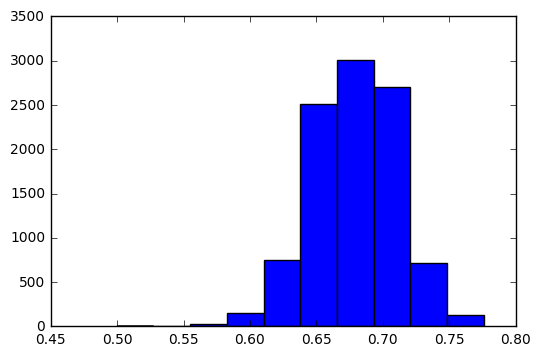In :
logit = lambda p: np.log(p/(1-p))
invlogit = lambda x: 1./(1 + np.exp(-x))

n_iterations = 10000

# Initialize trace for parameters
trace = np.empty(n_iterations+1)

# Set initial values
trace = 0.5
# Calculate joint posterior for initial values
current_log_prob = calc_posterior(trace)

# Initialize acceptance counts
accepted = 0

for i in range(n_iterations):

if not i%1000: print('Iteration %i' % i)

# Grab current parameter values
d_current = trace[i]

# Propose new value for d
d_prop = invlogit(logit(d_current) + np.random.uniform(-1, 1))

# Calculate log posterior with proposed value
proposed_log_prob = calc_posterior(d_prop)

# Log-acceptance rate
alpha = proposed_log_prob - current_log_prob

# Test proposed value
if np.log(np.random.random()) < alpha:
# Accept
trace[i+1] = d_prop
current_log_prob = proposed_log_prob
accepted += 1
else:
# Reject
trace[i+1] = trace[i]

Iteration 0
Iteration 1000
Iteration 2000
Iteration 3000
Iteration 4000
Iteration 5000
Iteration 6000
Iteration 7000
Iteration 8000
Iteration 9000

In :
plt.plot(trace)

Out:
[<matplotlib.lines.Line2D at 0x111671588>]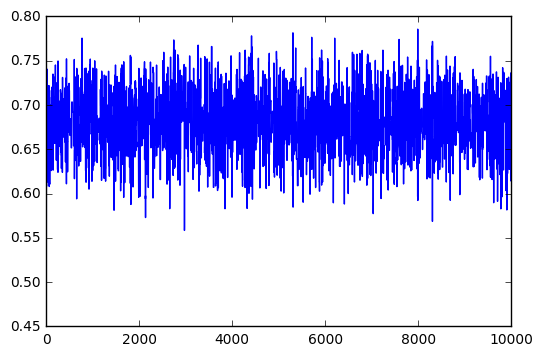# Question 4¶

Carlin (1992) considers a Bayesian approach to meta-analysis, and includes the following examples of 22 trials of beta-blockers to prevent mortality after myocardial infarction. These data are given below.

In one possible random effects model we assume the true baseline mean (on a log-odds scale) $m_i$ in a trial $i$ is drawn from some population distribution. Let $r^C_i$ denote number of events in the control group in trial $i$, and $r^T_i$ denote events under active treatment in trial $i$. Our model is:

\begin{aligned} r^C_i &\sim \text{Binomial}\left(p^C_i, n^C_i\right) \\ r^T_i &\sim \text{Binomial}\left(p^T_i, n^T_i\right) \\ \text{logit}\left(p^C_i\right) &= \mu_i \\ \text{logit}\left(p^T_i\right) &= \mu_i + \delta \\ \mu_i &\sim \text{Normal}(m, s). \end{aligned}

In this case, we want to make inferences about the population effect $m$, and the predictive distribution for the effect $\delta_{\text{new}}$ in a new trial.

This particular model uses a random effect for the population mean, and a fixed effect for the treatment effect. There are 3 other models you could fit to represent all possible combinations of fixed or random effects for these two parameters.

Build all 4 models to estimate the treatment effect in PyMC3 and

1. use convergence diagnostics to check for convergence in each model
2. use posterior predictive checks to compare the fit of the models
3. use DIC to compare the models as approximations of the true generating model

Which model would you select and why?

In :
r_t_obs = np.array([3, 7, 5, 102, 28, 4, 98, 60, 25, 138, 64,
45, 9, 57, 25, 33, 28, 8, 6, 32, 27, 22])
n_t_obs = np.array([38, 114, 69, 1533, 355, 59, 945, 632, 278,1916,
873, 263, 291, 858, 154, 207, 251, 151, 174, 209, 391, 680])
r_c_obs = np.array([3, 14, 11, 127, 27, 6, 152, 48, 37, 188, 52,
47, 16, 45, 31, 38, 12, 6, 3, 40, 43, 39])
n_c_obs = np.array([39, 116, 93, 1520, 365, 52, 939, 471, 282, 1921, 583, 266,
293, 883, 147, 213, 122, 154, 134, 218, 364, 674])
N = len(n_c_obs)

In :
with pm.Model() as fixed:

delta = pm.Normal('delta', 0, sd=10000)
mu = pm.Normal('mu', 0, sd=10000)

theta_t = pm.invlogit(mu + delta)*np.ones(N)
theta_c = pm.invlogit(mu)*np.ones(N)

treat = pm.Binomial('treat', n_t_obs, theta_t, observed=r_t_obs)
control = pm.Binomial('control', n_c_obs, theta_c, observed=r_c_obs)

In :
with fixed:
fixed_trace = pm.sample(5000, njobs=2)

Assigned NUTS to delta
Assigned NUTS to mu
100%|██████████| 5000/5000 [00:05<00:00, 890.16it/s]

In :
pm.forestplot(fixed_trace[-1000:], varnames=['delta', 'mu'])

Out:
<matplotlib.gridspec.GridSpec at 0x1143426a0>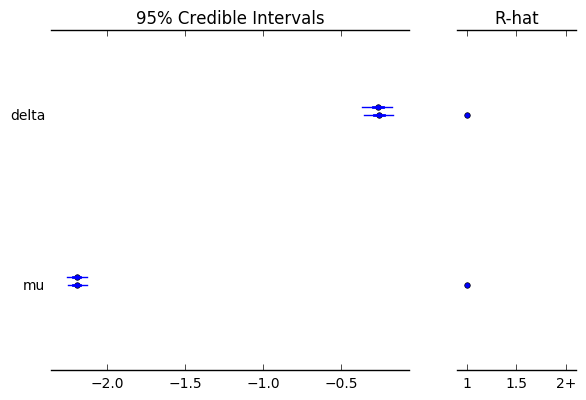In :
with pm.Model() as random_mean:

delta = pm.Normal('delta', 0, sd=10000)
m_mu = pm.Normal('m_mu', 0, sd=10000)
sigma_mu = pm.Uniform('sigma_mu', 0, 1000)
mu = pm.Normal('mu', m_mu, sd=sigma_mu, shape=N)

theta_t = pm.invlogit(mu + delta)*np.ones(N)
theta_c = pm.invlogit(mu)*np.ones(N)

treat = pm.Binomial('treat', n_t_obs, theta_t, observed=r_t_obs)
control = pm.Binomial('control', n_c_obs, theta_c, observed=r_c_obs)


In :
with random_mean:
random_mean_trace = pm.sample(5000, njobs=2)

Assigned NUTS to delta
Assigned NUTS to m_mu
Assigned NUTS to sigma_mu_interval_
Assigned NUTS to mu
100%|██████████| 5000/5000 [00:30<00:00, 164.76it/s]

In :
pm.forestplot(random_mean_trace[-1000:], varnames=['delta', 'm_mu', 'sigma_mu', 'mu'])

Out:
<matplotlib.gridspec.GridSpec at 0x124161320>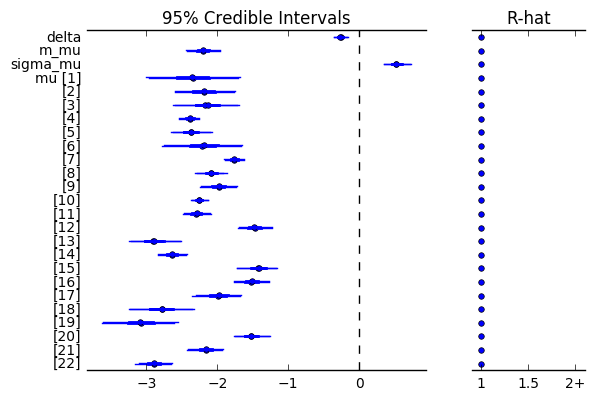In :
with pm.Model() as random_treat:

mu = pm.Normal('mu', 0, sd=10000)
m_delta = pm.Normal('m_delta', 0, sd=10000)
sigma_delta = pm.Uniform('sigma_delta', 0, 1000)
delta = pm.Normal('delta', m_delta, sd=sigma_delta, shape=N)

theta_t = pm.invlogit(mu + delta)*np.ones(N)
theta_c = pm.invlogit(mu)*np.ones(N)

treat = pm.Binomial('treat', n_t_obs, theta_t, observed=r_t_obs)
control = pm.Binomial('control', n_c_obs, theta_c, observed=r_c_obs)

In :
with random_treat:
random_treat_trace = pm.sample(5000, njobs=2)

Assigned NUTS to mu
Assigned NUTS to m_delta
Assigned NUTS to sigma_delta_interval_
Assigned NUTS to delta
100%|██████████| 5000/5000 [00:46<00:00, 106.88it/s]

In :
pm.forestplot(random_treat_trace[-1000:], varnames=['delta', 'm_delta', 'sigma_delta', 'mu'])

Out:
<matplotlib.gridspec.GridSpec at 0x1267382e8>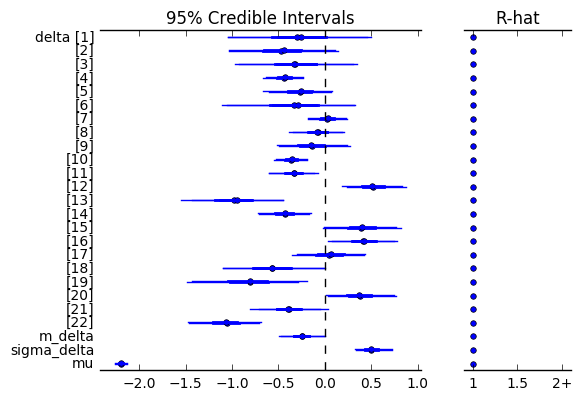In :
with pm.Model() as random:

m_mu = pm.Normal('m_mu', 0, sd=10000)
sigma_mu = pm.Uniform('sigma_mu', 0, 1000)
mu = pm.Normal('mu', m_mu, sd=sigma_mu, shape=N)

m_delta = pm.Normal('m_delta', 0, sd=10000)
sigma_delta = pm.Uniform('sigma_delta', 0, 1000)
delta = pm.Normal('delta', m_delta, sd=sigma_delta, shape=N)

theta_t = pm.invlogit(mu + delta)*np.ones(N)
theta_c = pm.invlogit(mu)*np.ones(N)

treat = pm.Binomial('treat', n_t_obs, theta_t, observed=r_t_obs)
control = pm.Binomial('control', n_c_obs, theta_c, observed=r_c_obs)

In :
with random:
random_trace = pm.sample(5000, njobs=2)

Assigned NUTS to m_mu
Assigned NUTS to sigma_mu_interval_
Assigned NUTS to mu
Assigned NUTS to m_delta
Assigned NUTS to sigma_delta_interval_
Assigned NUTS to delta
100%|██████████| 5000/5000 [00:47<00:00, 106.19it/s]

In :
pm.forestplot(random_trace[-1000:], varnames=['delta', 'm_delta', 'sigma_delta',
'mu', 'm_mu', 'sigma_mu'])

Out:
<matplotlib.gridspec.GridSpec at 0x12d7ac860>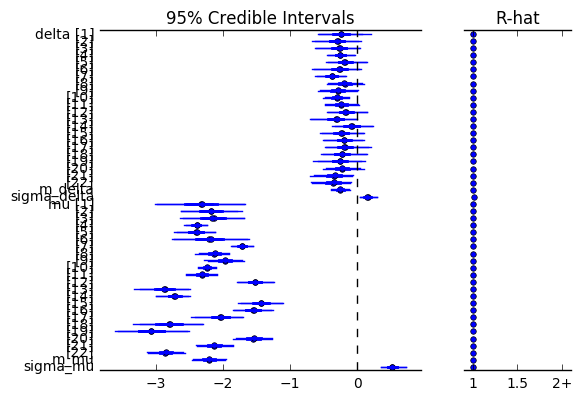In :
dic = {}

with random:
dic['random'] = pm.dic(random_trace)

with fixed:
dic['fixed'] = pm.dic(fixed_trace)

with random_mean:
dic['random_mean'] = pm.dic(random_mean_trace)

with random_treat:
dic['random_treat'] = pm.dic(random_treat_trace)

dic

Out:
{'fixed': 585.77413217782919,
'random': 412.91279623664684,
'random_mean': 413.10790182084617,
'random_treat': 552.16711937961054}

This implies that the fully random or the random mean models are preferred.

### Posterior predictive checks¶

In :
ppc_random = pm.sample_ppc(random_trace, model=random, samples=500)

100%|██████████| 500/500 [00:05<00:00, 91.84it/s]


I'm calculating Bayesian p-values here, but could do it graphically as well.

In :
from scipy.stats import percentileofscore

p = [percentileofscore(s, o).round(2) for s,o in zip(ppc_random['treat'].T, r_t_obs)]
p += [percentileofscore(s, o).round(2) for s,o in zip(ppc_random['control'].T, r_c_obs)]

plt.hist(p)
plt.xlabel('percentile')
plt.ylabel('frequency');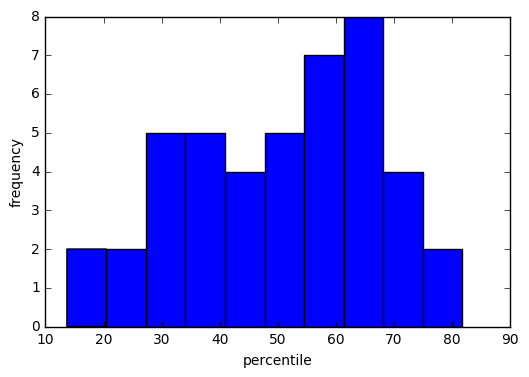In :
ppc_random_mean = pm.sample_ppc(random_mean_trace, model=random_mean, samples=500)

100%|██████████| 500/500 [00:04<00:00, 110.70it/s]

In :
p = [percentileofscore(s, o).round(2) for s,o in zip(ppc_random_mean['treat'].T, r_t_obs)]
p += [percentileofscore(s, o).round(2) for s,o in zip(ppc_random_mean['control'].T, r_c_obs)]

plt.hist(p)
plt.xlabel('percentile')
plt.ylabel('frequency');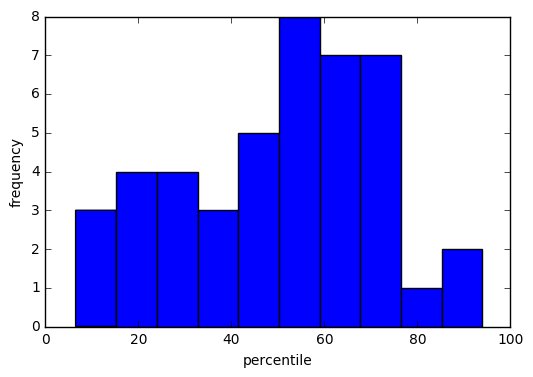There is no evidence of lack of fit in either of the preferred models.